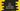# C++ program to print the current date, day and time## C++ program to print the current date, day and time:

In this post, we will learn how to print the current date, day and time. Dealing with time is already defined in C++ and we can easily find out the current time details using a couple of functions.

This post will show you how to print the current date, day and time in C++.

### date, day and time printing in C++:

#### time():

This is the main function. It will return the current time. The return type of this function is of type time_t. This type is used to store current date time related data.

#### localtime():

It is the second function that we need to use. It takes one argument of type time_t and fills one structure with the time value for the argument passed. It expressed in local timezone.

#### asctime():

This is another function to convert the return structure of localtime to human readable format. This function takes one structure like the one we got in localtime as argument and and converts it to a C-string containing a human readable version of date and time.

It returns one string with the below format :

``Www Mmm dd hh:mm:ss yyyy``

Here,

``````Www : It is the weekday
Mmm : It is month in letters
dd : Day of the month
hh : Hour
mm : Minutes
ss : Seconds
yyyy : Year``````

### C++ program :

Below is the complete C++ program that shows how to print the current date time:

``````#include <iostream>
#include <ctime>
using namespace std;

string getCurrentDateTime()
{
time_t tt;
struct tm *st;

tt = time(NULL);
st = localtime(&tt);
return asctime(st);
}

int main()
{
cout << "Current Date time : " << getCurrentDateTime();
}``````

Here,

• getCurrentDateTime method is used to get the current date time string. It returns one string.
• tt is a variable of type time_t to hold the return value of time().
• st is a variable to hold the value of localtime.
• asctime is called on st to get the final result.
• We can also write time(&tt) instead of tt = time(NULL)

If you run this, it will print the current date time as like below :

``Current Date time : Sat Nov 28 12:28:00 2020``